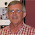## Tuesday, October 30, 2012

### Some Properties of Non-linear Least Squares

You probably know that when we have a regression model that is non-linear in the parameters, the Non-Linear Least Squares (NLLS) estimator is generally biased, but it's weakly consistent. This is the case even if the model has non-random regressors and an additive error term that satisfies all of the usual assumptions.

In addition, even if the model’s errors are normally distributed, the NLLS estimator will have a sampling distribution that is non-normal in finite samples, and the usual t-statistics will not be Student-t distributed in finite samples.

In this post I'll illustrate these, and some other results, by using a simple Monte Carlo experiment.

As the sample size grows without limit, eventually the bias of the NLLS estimator vanishes – it's an asymptotically unbiased estimator. Generally, its variance also converges to zero, meaning that the estimator is mean square consistent as well as weakly consistent.

Also, as the sample size grows, eventually the sampling distribution of the NLLS estimator becomes (asymptotically) normal, due to the central limit theorem, and the t-statistics have their usual asymptotic properties – in particular, they will also be normally distributed.

I've used the EViews workfile and program file, available on the code page for this blog, to illustrate the above results for this very simple non-linear regression model:

yi = β1 + (β2x2i)β1 + εi  ;   εi ~ i.i.d. N[0 , σ2]       ;     i = 1, 2, …., n .

The values assigned to the parameters are β1 = 1.0; β2 = 2.0; and σ = 1.0. Using 5,000 replications in the Monte Carlo experiment, the following results were obtained with respect to the NLLS estimator of  (see the READ_ME text-object in the EViews workfile):

n           Bias(b2)           Var.(b2)

10 0.22704 1.00489
25 0.07956 0.22524
50 0.03926 0.08556
100 0.01077 0.02190
250 0.00348 0.00692
500 0.00144 0.00404
1,000 0.00148 0.00230
5,000 0.00049         0.00039
10,000 0.00011 0.00012

(Here, b2 is the NLLS estimator for β2.)

These results illustrate the bias and the mean-square consistency of the estimator. Although a sample of size 500 appears to be sufficient for the consistency of the estimator to become apparent, the convergence is not so rapid when it comes to the normality of the sampling distributions for the estimator itself and for the associated t-statistic (let's call it t2) for testing H0: β2 = 2. (So, the null hypothesis is true.) This is illustrated in the following charts.
The non-normality of the sampling distribution of b2 is obvious.

It's clear that the sampling distribution of t2, the t-statistic, is neither t-distributed nor normally distributed.

The non-normality of the sampling distribution of b2 is quite clear from the skewness coefficient, and the p-value associated with the Jarque-Bera test statistic.

The non-normality of the sampling distribution of t2 is really obvious from the skewness coefficient and the  very small p-value for the Jarque-Bera test statistic.

Using the Jarque-Bera test, we can't reject the hypothesis that the sampling distribution of b2 is normal, when n = 10,000.

Finally,we cannot reject the hypothesis that the sampling distribution of t2 is standard normal when n = 10,000.

As we can see, even when we have a very simple non-linear regression model, we may need a VERY large sample size before the asymptotics fully come into play.

This last point is pretty important. Students often ask: "How large does the sample size have to be before we can rely on results that are valid only asymptotically?" The correct answer (and it's not a "cop-out") is: "It depends."

It depends on the particular problem, the data values, and often the true values of the unobservable parameters. So, be cautious!

© 2012, David E. Giles

1.Hi, Giles,

I think this is the case that it is very easy to conflate statiscal signficance with practical significance.

See, for example, in the last case, with n=10.000 for t2, the p-value is indeed small, but that doesn't really matter.

What we want to know is if the departure from normality is big enough so that it wouldn`t be ok to use a t test. That is, we want to know if the departures from Skewness and Curtosis are big enough that the normal or t distribution would be a bad approximation.

The p-value will not tell us how big the departure is. See, even if the distribution were normal Skew=0,0000001, Kurt=3,000001, if we take a huge enough sample, we could get p<0,0000001.

So what we ought to do in this case is to see if the suggested deviation in Skewness and Kurtosis provided by the data is big enough to practical matters.

Some people say that a Skewness below 0.3 and Kurtosis between 2,5-3,5 could be ok form most purposes.

So, in that case, n=500 could aready be enough a sample, even though we would still reject perfect normality.

Best

Carlos

1.Carlos - fair point.

Of course, my final point still stands - there are lots of situations where very, very large sample sizes are needed before the asymptotics "kick in".

2.Yeah, I agree with you, I think one of the most problematic cases are the robust standard errors!

Everyone in applied econometrics uses them today, even with really small samples, with no concern about when would asymptotics really kick in!

Best

Carlos

3.Carlos - absolutely correct. On this point, see
http://d.repec.org/n?u=RePEc:nbr:nberwo:18478&r=ecm

2.Very interesting! IV estimators are of course another important case in point.

1.Thanks - yes, you're absolutely right.